# Using Place Value Mats for Multiplication & Division

Not all kids learn the same way. And not all kids just get the idea multiplying and dividing. I know that my girls each had their own way of learning and we used different strategies to with of them.

I have one that really is a hands-on learner. She does best when she can see how things all work together. Not just the memorizing of math facts.

Today’s multiplication and division help chart are great for helping with hands-on learning. You can learn about making groups and multiplying groups in a place value setting.Each of these mats is very basic to use for multiplication and division. The mats each have columns and the place value is shown above. They come in one & tens; one, tens & hundreds; one, tens, hundreds & thousands.

I like to laminatethese or put them in sheet protectors. I often store them in sheet protectorsin a help binder. I put the multiplication and division mats back to back in the sheet protectors. So that tens and ones are both together.## How we use these multiplication and division printables

Before I start on using maths like these I always reminded my girls that you can’t have more than 9 numbers in a place value line. I would pull out the place value mats and show them the 9 spots. I would then say if we have more than 9, they all become a one and move up to the next place value. The same goes for when you borrow. If you borrow from another column you take 10, not just one.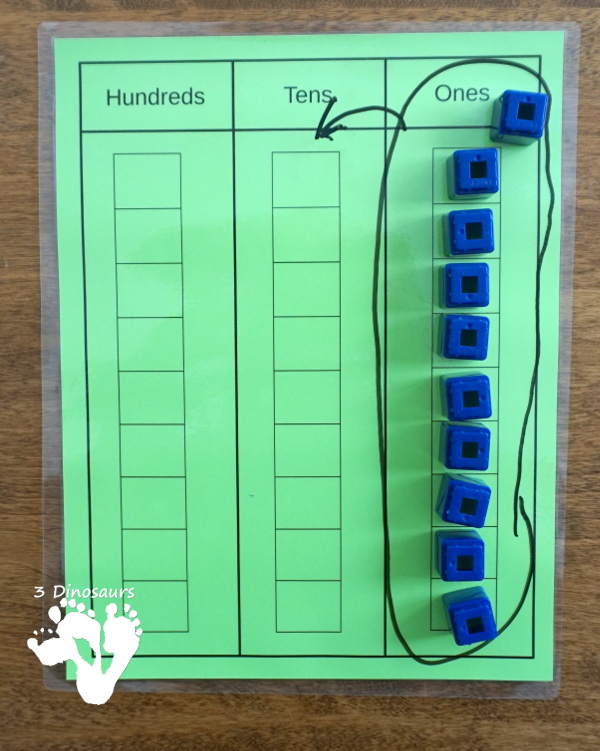I always start with multiplication and groups of things. I use the flash cards for 3×3 is 3 groups of three. I prep a mat and unifix cubeslike the image below.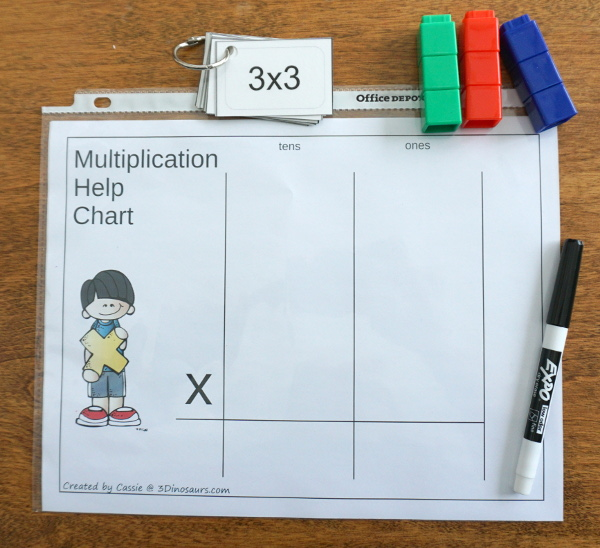When you put the three groups of three together it just makes nine. You can see how the different colors of unifix cubesstill show the groups in multiplying the small numbers.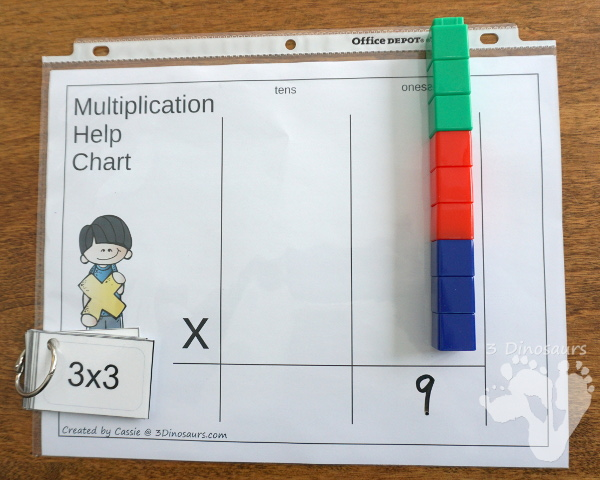This works on any group of numbers. You can see below in the next few pictures how we did this for 8×7 flashcard.

I set up the area with the card and the unifix cubesto do the problem. You can also have kids set this up as well.Next, I had them put the unifix cubestogether in groups of ten. If they made ten they placed them in the ten column. If they had any less then ten it stayed in the one’s column.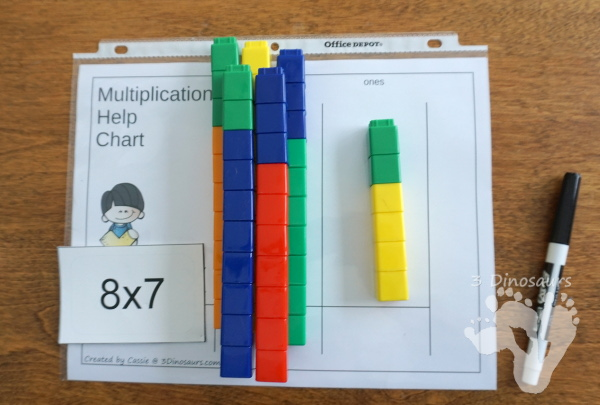We moved made then ten to just one cube and put the extra cubes above. We wrote in the answer for the multiplication.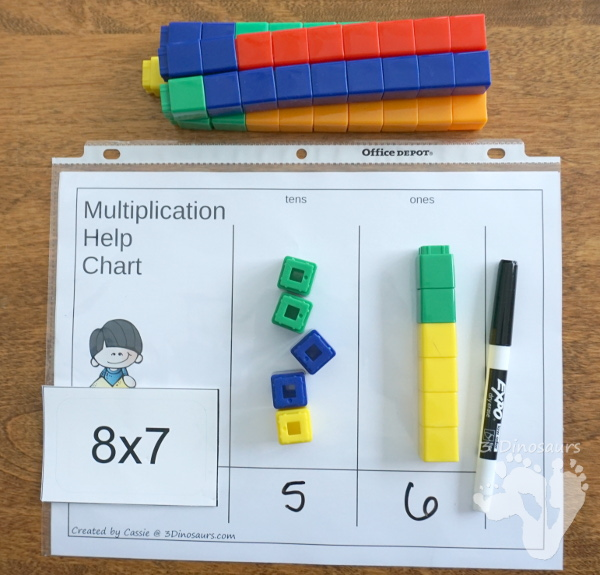This hands-on approach always works for bigger multiplication problems as well. I find this a great way to work with those that need some extra help with math and might need to see how things work.Next we work up to Division. I find that division is always harder than multiplication. The wording can be hard at times and there are so many ways to say it. If you have taught multiplication with place value then using it for the division will help as well.

I always start with flash cards and easier ones to start. Below I show you how we did 40 divided by 8. You can see how I set up the area. I have the 4 in the tens and the extra unifix cubesabove.We look a the tens column and see if we can make any groups of 8. Nope, we can’t so we have to move the 4 in the tens to the ones column.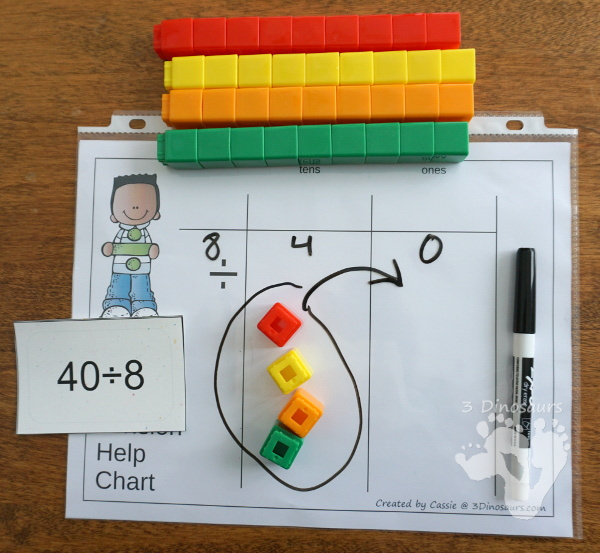I show that we moved and have 4 groups of 10 in the ones column.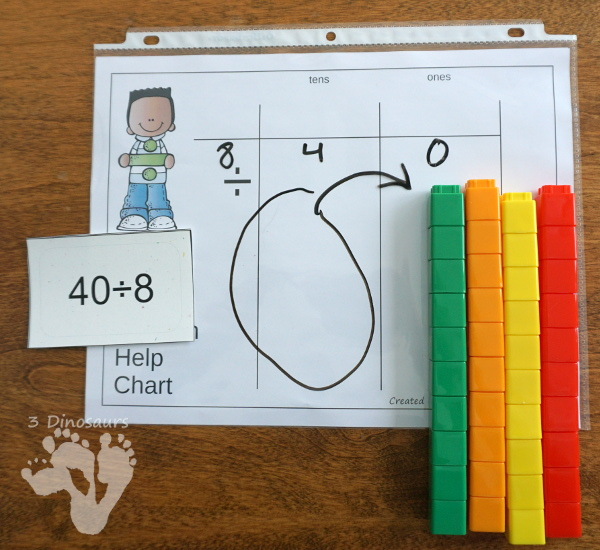Then we make groups of eight. I always stand them up so it looks like just one of each. This is a good way to see how many groups we made from the unifix cubes.We then take one cube on each column to show the answer to our flash card. I keep the other cubes close by so we can still see it is groups of 8 that we were looking for.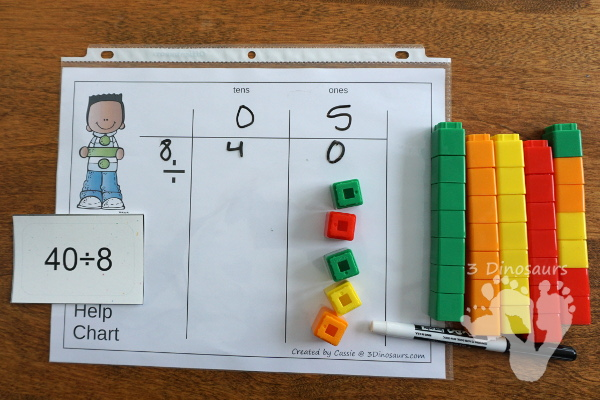Now nothing says you have to use cubs. You can just use the sheet in a sheet protectorsand dry erase marker. Then just write in the answer as you need them.## What you will find in these printables

• Multiplication and division mats with a place value chart
• 3 Charts: one & tens; one, tens & hundreds; one, tens, hundreds & thousands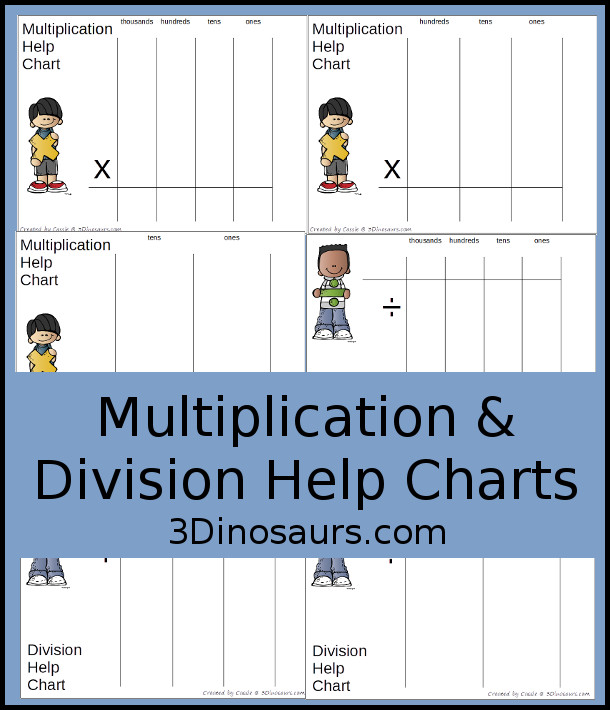Check out these other Multiplication and Divison activities:

Cassie – 3Dinosaurs.com